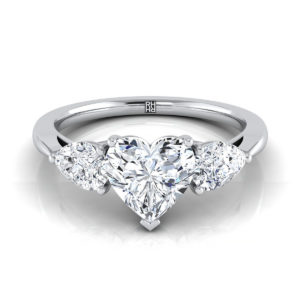# How to Determine the Carat Weight of a Heart Cut Diamond?The approximate carat weight of the diamond in your heart solitaire diamond ring can be calculated using measures like width, depth, and length. The formula for calculating the carat weight using these attributes is:

Carat Weight = Length x Width x Depth x 0.006

Length: It is the distance from the pointed end to the farthest point on the cleft of the stone.

Width: This is the measure of the widest part of the stone.

Depth: Depth is the distance between the lowest point at the bottom of the heart stone to its topmost facet.

Example

If you have a heart cut diamond with length and width 5mm each, and depth3mm, then the carat weight would be as follows:

Carat Weight = Length x Width x Depth x 0.006

Carat Weight = 5mm x 5mm x 3mm x 0.006 = 0.45 ct

While calculating this, the value obtained would not be very precise, as you can expect an estimation error of +/- 10 to 15%. In the above case, the carat weight of the diamond could be anywhere between 0.40 to 0.50 ct.